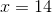Praxis Math : How to reason and solve one-variable equations and inequalities

Example Questions

Example Question #1 : How To Reason And Solve One Variable Equations And Inequalities

Solve for.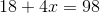Cannot be determinedExplanation:

In order to solve for the variable,, we need to isolate it on the left side of the equation. We will do this by reversing the operations done to the variable by performing the opposite of each operation on both sides of the equation.

Let's begin by rewriting the given equation.Subtractfrom both sides of the equation.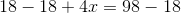Simplify.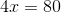Divide both sides of the equation by.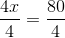Solve.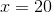Example Question #2 : How To Reason And Solve One Variable Equations And Inequalities

Solve for.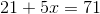Cannot be determinedExplanation:

In order to solve for the variable,, we need to isolate it on the left side of the equation. We will do this by reversing the operations done to the variable by performing the opposite of each operation on both sides of the equation.

Let's begin by rewriting the given equation.Subtractfrom both sides of the equation.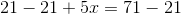Simplify.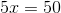Divide both sides of the equation by.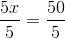Solve.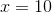Example Question #3 : How To Reason And Solve One Variable Equations And Inequalities

Solve for.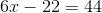Cannot be determinedExplanation:

In order to solve for the variable,, we need to isolate it on the left side of the equation. We will do this by reversing the operations done to the variable by performing the opposite of each operation on both sides of the equation.

Let's begin by rewriting the given equation.Addto both sides of the equation.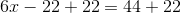Simplify.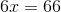Divide both sides of the equation by.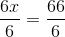Solve.Example Question #4 : How To Reason And Solve One Variable Equations And Inequalities

Solve for.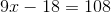Cannot be determinedExplanation:

In order to solve for the variable,, we need to isolate it on the left side of the equation. We will do this by reversing the operations done to the variable by performing the opposite of each operation on both sides of the equation.

Let's begin by rewriting the given equation.Addto both sides of the equation.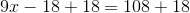Simplify.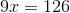Divide both sides of the equation by.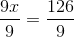Solve.